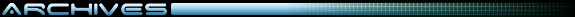Game Programming and Development Tools

arrgG! whats with this?? (circular algorithms)... – Realm Master

Realm MasterMember

Posts: 1971
From: USA
Registered: 05-15-2005
aaahhh!!! i cant figure this out!!!

Equasion for circular distance: (D = (square root of)[(x1 - x2)^2 + (y1 - y2)^2]

got it, but here's MY delema: i want it so that when i "rotate" one point in my program, the other point "rotates too" such as like this.

two points lie on the same line (or sorta) the "base" point roatates, and the far point moves so that it lies on a circle (depicting the greatest distance from the base point) always being the same distance from the base point.

in otherwords: i want a joint to work, picture it like your elbow and hand: when you rotate your elbow, your hand moves in an arch. I want it like that, only, it can go in a whole circle because... bah! you get the idea...

can i have a little help?

(grumbles: I gotta learn trig.... and more geomentry)

------------------
(yes, i know im stupid)

Blessed are those who suffer for doing what is right.
The kingdom of hevan bleongs to them.-Matthew 5:10

PM ME YOUR DESCRIPTION OF ME! ILL PUT IT HERE!

Here's all the comments!

vincentMember

Posts: 129
From: Amersfoort, the Netherlands
Registered: 12-23-2002
Well, if I understand what you mean you do there are several ways to go about... First of all, how did you define the points from the base point... for example, if you defined them by a distance and an angle, its quite simple...

 p1x = cos(angle + rotation) * distance + basexp1y = sin(angle + rotation) * distance + baseyp2x = cos(angle2 + rotation) * distance + basexp2y = sin(angle2 + rotation) * distance + basey

Then its just a matter of changing the rotation.

However, if the points are defined by an x and a y, you'll need to let good 'ole matrix multiplication do its work:

http://mathworld.wolfram.com/RotationMatrix.html

which basically says that:

 [cos(angle) sin(angle)] [x y] * [ ] [-sin(angle) cos(angle)]

which means that

x' = x * cos(angle) + y * -sin(angle)
y' = x * sin(angle) + y * cos(angle)

I think.. though it has been a while ago... This basically means that if you have a point (1, 2) and you want to rotate it say, 45 degrees:

x' = 1 * cos(45) + 2 * -sin(45)
y' = 1 * sin(45) + 2 * cos(45)

This always rotates around the 0 point... so, you'll need to substract some first, and add later... anyway, I hoped it helped a bit.. note that most programming languages use radiants.. so 45 degrees would be PI/4.... anyway... hope it helps, and I hope its correct, cause it has been a while.

[This message has been edited by vincent (edited October 14, 2005).]

Realm MasterMember

Posts: 1971
From: USA
Registered: 05-15-2005
dang, i HATE not kowing this stuff...

thanks vincent!

edit:

gaaa! I cant seem to get it to work...

bah! All of my other friends are enjoying their day, and i spend it (doing something i love to do, mind you) working on somehting that ill never finish. I Always, ALWAYS, run into something i dunno how to do...

*sigh*... firemaker... how do you DO it??! (being a child prodegy and all)

------------------
(yes, i know im stupid)

Blessed are those who suffer for doing what is right.
The kingdom of hevan bleongs to them.-Matthew 5:10

PM ME YOUR DESCRIPTION OF ME! ILL PUT IT HERE!

Here's all the comments!

[This message has been edited by Realm Master (edited October 14, 2005).]

vincentMember

Posts: 129
From: Amersfoort, the Netherlands
Registered: 12-23-2002
Alright.. lemme try putting it into semi-code.

 function rotate(orginX, orginY, objectX, objectY, rotation) { x = objectX - orginX; //since we only rotate around the orgin we need to y = objectY - orginY; //substract the point we want to rotate around nx = x * cos(rotation) + y * (-sin(rotation)) // do the rotation ny = x * sin(rotation) + y * cos(rotation) nx = nx + orginX; // add the orgin again to move the point back ny = ny + orginY; // to where it belongs return (nx, ny)}

Then ofcourse we might want to know what the rotation is of a point from the given orgin. Note that most programming languages have a tan2(x,y) function.. which does exactly the same thing as this here under, except for the orgin thing. If it does, I suggest you use it. Then you only need to do the orgin substraction thing.

 function getRotation(orginX, orginY, px, py) { x = px - orginX; // get the relative difference, making orgin = (0,0) y = py - orginY; r = 0; if (y != 0) { // avoid devide by 0. Besides, if the Y = 0, degrees = 0 r = atan(x/y) } // is the X is < 0, the TAN would return the results - 180 degrees // because a TAN only returns -90 to 90 degrees and doesn't really // bother to use 360 degrees. So we need to do that manually. if (x < 0) { r = r + 180 degrees (= PI) // and we're good. } if (r < 0) { // TAN can return negative values, we only want positive // so add 360 degrees. r = r + 360 degrees (= 2 * PI) } return r;}

Well, I hope it helps a bit. I haven't tested it.. so it might be wrong, but it might give you some clue into what direction you are looking.